# Multiple-correlation coefficient

(diff) ← Older revision | Latest revision (diff) | Newer revision → (diff)

A measure of the linear dependence between one random variable and a certain collection of random variables. More precisely, if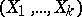is a random vector with values in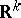, then the multiple-correlation coefficient betweenand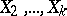is defined as the usual correlation coefficient between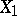and its best linear approximation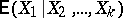relative to, i.e. as its regression relative to. The multiple-correlation coefficient has the property that if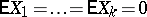and if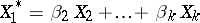is the regression ofrelative to, then among all linear combinations ofthe variable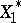has largest correlation with. In this sense the multiple-correlation coefficient is a special case of the canonical correlation coefficient (cf. Canonical correlation coefficients). Forthe multiple-correlation coefficient is the absolute value of the usual correlation coefficient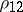betweenand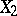. The multiple-correlation coefficient betweenandis denoted by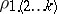and is expressed in terms of the entries of the correlation matrix,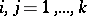, by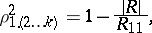whereis the determinant ofandis the cofactor of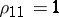; here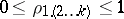. If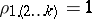, then, with probability,is equal to a linear combination of, that is, the joint distribution of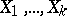is concentrated on a hyperplane in. On the other hand,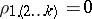if and only if, that is, ifis not correlated with any of. To calculate the multiple-correlation coefficient one can use the formula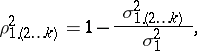where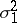is the variance ofand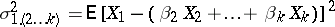is the variance ofwith respect to the regression.

The sample analogue of the multiple-correlation coefficientiswhereand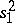are estimators ofandbased on a sample of size. To test the hypothesis of no relationship, the sampling distribution of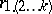is used. Given that the sample is taken from a multivariate normal distribution, the variable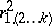has the beta-distribution with parameters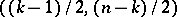if; if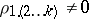, then the distribution ofis known, but is somewhat complicated.